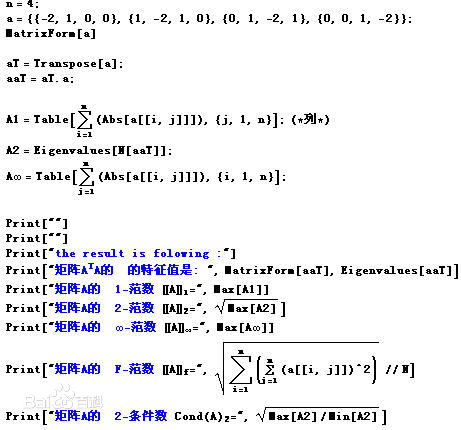# 向量与矩阵范数

## 向量范数

### 概念

1. 正定性：f(x)0xRn$f(x)\geq 0，\forall x\in R^n$，等号当且仅当 x=0 $x=0$时候成了。

• 正齐次性： f(αx)=|α|×f(x)xRnαR $f(\alpha x) = |\alpha|\times f(x)，\forall x\in R^n，\forall \alpha \in R$
• 次可加性（三角不等式）： f(x+y)f(x)+f(y) $f(x+y)\leq f(x)+f(y)$
• 例如：

xp=(i=1n|xi|p)1p,p[1,)Rn

### 常见的范数

这里以 Cn $C^n$空间为例， Rn $R^n$空间类似。
最常用的范数就是p-范数。若 x=[x1,x2,,xn]T $x=[x_1,x_2,\dots,x_n]^T$，那么

xp=(|x1|p+|x2|p++|xn|p)1p

可以验证p-范数确实满足范数的定义。其中三角不等式的证明不是平凡的，这个结论通常称为闵可夫斯基(Minkowski）不等式。

当p取1，2，∞的时候分别是以下几种最简单的情形：
1-范数： x1=x1+x2++xn $║x║_1=│x_1│+│x_2│+…+│x_n│$
2-范数： x2=(x12+x22++xn2)1/2 $║x║_2=(│x_1│^2+│x_2│^2+…+│x_n│^2)^{1/2}$
∞-范数(最大范数)： x=max(x1x2xn $║x║_∞=\max(│x_1│，│x_2│，…，│x_n│）$

其中2-范数就是通常意义下的距离。

### 范数的性质

(1)连续性
f $f$Rn$R^n$上的任意一个范数，则 f $f$关于x$x$的每个分量是连续的。

(2)等价性
s $\|\cdot\|_s$ t $\|\cdot\|_t$ Rn $R^n$上的任意两个范数，则存在常数 c1 $c_1$ c2 $c_2$，使得对任意的 xRn $x\in R^n$

c1xsxtc2xs

(3)不等式

对于这些常用的范数有以下不等式： xx2x1n12x2nx $║x║∞ ≤ ║x║_2 ≤ ║x║_1 ≤ n^{1\over 2}║x║_2 ≤ n║x║_∞$
另外，若p和q是赫德尔（Hölder）共轭指标，即1/p+1/q=1，那么有赫德尔不等式：

|<x,y>|=||xHy|xpyq

当p=q=2时就是柯西-许瓦兹（Cauchy-Schwarz）不等式。

(4)向量序列的收敛性

limkx(k)=xlimkx(k)x=0

## 矩阵范数

### 概念

一般来讲矩阵范数除了正定性，齐次性和三角不等式之外，还规定其必须满足相容性： XYXY $║XY║≤║X║║Y║$

所以矩阵范数通常也称为相容范数。

如果 α $║·║_α$是相容范数，且任何满足 βα $║·║_β≤║·║_α$的范数 β $║·║_β$都不是相容范数，那么 α $║·║_α$称为极小范数。对于 n $n$阶实方阵（或复方阵）全体上的任何一个范数$║·║$，总存在唯一的实数 k>0 $k>0$，使得 k× $k\times ║·║$是极小范数。
注：如果不考虑相容性，那么矩阵范数和向量范数就没有区别，因为 m×n $m\times n$矩阵全体和 mn $mn$维向量空间同构。引入相容性主要是为了保持矩阵作为线性算子的特征，这一点和算子范数的相容性一致，并且可以得到Mincowski定理以外的信息。

### 常见的矩阵范数

满足以上设定的矩阵范数可以有多种。由于它们都是定义在 Mm,n(K) $\mathcal{M}_{m, n}(\mathbb{K})$这个有限维向量空间上的范数，所以实质上是等价的。常见的矩阵范数通常是在矩阵的应用中自然定义或诱导的范数。

向量范数诱导的矩阵范数

考虑从向量空间 V=Km $V = \mathbb{K}^m$映射到 W=Kn $W = \mathbb{K}^n$的所有线性映射的构成的空间： Lm,n(K) $\mathcal{L}_{m, n}(\mathbb{K})$。设 V $V$W$W$中分别装备了两个向量范数 V $\| \cdot \|_V$ W $\| \cdot \|_W$，则可以定义 Lm,n(K) $\mathcal{L}_{m, n}(\mathbb{K})$上的算子范数 L $\| \cdot \|_\mathcal{L}：$

ALm,n(K) $\forall A \in \mathcal{L}_{m, n}(\mathbb{K})$

AL=max{A(x)W;xV,xV1}

而给定了基底后，每个从V映射到W的线性映射都可以用一个m \times n的矩阵来表示，所以同样地可以定义 Mm,n(K) $\mathcal{M}_{m, n}(\mathbb{K})$上的非负映射 M $\| \cdot \|_\mathcal{M}：$

AMm,n(K) $\forall A \in \mathcal{M}_{m, n}(\mathbb{K})$

AL=max{A(x)W;xV,xV1}

可以验证， M $\| \cdot \|_\mathcal{M}$满足矩阵范数的定义，因此是一个矩阵范数。这个矩阵范数被称为是由向量空间范数诱导的矩阵范数，可以看作是算子范数在由有限维向量空间之间线性映射组成的空间上的特例。如果 m=n $m = n$，所对应的矩阵空间就是n阶方块矩阵空间 Mn(K) $\mathcal{M}_{n}(\mathbb{K})$。这时可以验证，诱导范数 M $\| \cdot \|_\mathcal{M}$满足一致性条件。

p-范数诱导的矩阵范数

V $V$W$W$中装备的向量范数都是p-范数的时候，诱导的矩阵范数也称为矩阵的诱导p-范数。具体来说就是：

Ap=maxx0Axpxp=maxx0(ni=1|mj=1Aijxj|p)1/p(mi=1|xi|p)1/p

在p=1和 p= $p=\infty$的情况下，其范数可以以下方式计算：

A1=max1jni=1m|aij|A=max1imj=1n|aij|.

这些与矩阵的Schatten p-范数不同，也可以用 Ap $\left \| A \right \| _p$来表示。
当p = 2（欧几里德范数）且m = n（方阵）时，诱导的矩阵范数就是谱范数。矩阵A的谱范数是A最大的奇异值或半正定矩阵A*A的最大特征值的平方根：
A2=λmax(AA)

其中 A $A*$代表A的共轭转置。

矩阵元范数

这些向量范数将矩阵视为 m×n $m \times n$向量，并使用类似的向量范数。
举例说明，使用向量的p-范数，我们得到：

Ap=(i=1mj=1n|aij|p)1/p

注：不要把矩阵元p-范数与诱导p-范数混淆。

弗罗贝尼乌斯范数

p=2 $p = 2$，这称为弗罗贝尼乌斯范数（Frobenius norm）或希尔伯特-施密特范数（Hilbert–Schmidt norm），不过后面这个术语通常只用于希尔伯特空间。这个范数可用不同的方式定义：

AF=i=1mj=1n|aij|2=trace(AA)=i=1min{m,n}σ2i

这里 A $A*$表示 A $A$的共轭转置，σi$σi$ A $A$奇异值，并使用了迹函数。弗罗贝尼乌斯范数与Kn$K^n$上欧几里得范数非常类似，来自所有矩阵的空间上一个内积。
弗罗贝尼乌斯范范数是服从乘法的且在数值线性代数中非常有用。这个范数通常比诱导范数容易计算。

### 矩阵范数性质

矩阵范数的性质

(1)连续性：设 f $f$Rn×n$R^{n\times n}$上的任一矩阵范数，则 f $f$关于A$A$的每个分量是连续的。

(2)等价性：设 s $\|\cdot\|_s$ t $\|\cdot\|_t$ Rn×n $R^{n\times n}$上的任意两个矩阵范数，则存在常数 c1 $c_1$ c2 $c_2$，使得对任意的 ARn×n $A\in R^{n\times n}$

c1AsAtc2As

(3)
任何诱导的矩阵范数都满足此不等式
Aρ(A)

其中 ρ(A) $ρ(A)$ A $A$的谱半径。事实上，可以证明ρ(A)$ρ(A)$ A $A$的所有诱导范数的下界。
此外，我们有
limrAr1/r=ρ(A)$\lim_{r\rightarrow\infty}\|A^r\|^{1/r}=\rho(A)$
若A是对称矩阵，则 ρ(A)=A2 $ρ(A)=\|A\|_2$

算子范数的性质

定理：
$\|\cdot\|$ Rn $R^n$上的任一向量范数，其对应的算子范数也记为 $\|\cdot\|$，则有

AxA×x

定理：
$\|\cdot\|$是任一算子范数，则 ρ(A)A $ρ(A)\leq \|A\|$

定理：
对任意 ε>0 $\varepsilon > 0$，总存在一个算子范数 ε $\|\cdot\|_\varepsilon$，使得

ερ(A)+ε

这个图的计算可能有一些问题05-041万+09-182万+
06-041万+
09-232万+
08-1416万+
06-2416万+
04-081218
08-236158
02-057475
07-185万+
12-047324
05-299320
03-216899
12-304444
08-04389
02-071668
09-275万+
04-191万+
©️2020 CSDN 皮肤主题: 大白 设计师:CSDN官方博客